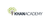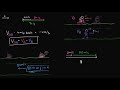# Class 11 Physics (India): Calculating relative velocity

Type product
Tijdsduur

## Class 11 Physics (India): Calculating relative velocityKhan Academy

Tip: laat jezelf inspireren, bekijk hier de video!### Beschrijving

How do we calculate the velocity of one object as seen by another object? In this video, we will intuitively figure out how to calculate relative velocities with some examples. We'll then write down the general expression for calculating relative velocity. Created by Mahesh Shenoy.

## Class 11 Physics (India)

Let's learn, practice, and master topics of class 11 physics (NCERT) starting with kinematics and then moving to dynamics with Newton's laws of motion, work, energy, and power. Let's then use these as the foundation to learn about centre of mass, rotational motion, gravitation, solids, fluids, thermodynamics, and oscillations and waves.

## Relative velocity in 1D

Let's understand and learn h…

Lees de volledige beschrijving

### Veelgestelde vragen

Er zijn nog geen veelgestelde vragen over dit product. Als je een vraag hebt, neem dan contact op met onze klantenservice.

Nog niet gevonden wat je zocht? Bekijk deze onderwerpen: Natuurkunde, Biologie, Chemie, Wildbeheer en PHP.

How do we calculate the velocity of one object as seen by another object? In this video, we will intuitively figure out how to calculate relative velocities with some examples. We'll then write down the general expression for calculating relative velocity. Created by Mahesh Shenoy.

## Class 11 Physics (India)

Let's learn, practice, and master topics of class 11 physics (NCERT) starting with kinematics and then moving to dynamics with Newton's laws of motion, work, energy, and power. Let's then use these as the foundation to learn about centre of mass, rotational motion, gravitation, solids, fluids, thermodynamics, and oscillations and waves.

## Relative velocity in 1D

Let's understand and learn how to solve problems involving relative motion and frames of reference when the motion happens on a straight line.

## Topic: Science

Learn about all the sciences, from physics, chemistry and biology, to cosmology and astronomy, across hundreds of videos, articles and practice questions.

Er zijn nog geen ervaringen.
• Bekijk gerelateerde producten mét ervaringen: Natuurkunde.

### Deel je ervaring

Heb je ervaring met deze cursus? Deel je ervaring en help anderen kiezen. Als dank voor de moeite doneert Springest € 1,- aan Stichting Edukans.

Er zijn nog geen veelgestelde vragen over dit product. Als je een vraag hebt, neem dan contact op met onze klantenservice.